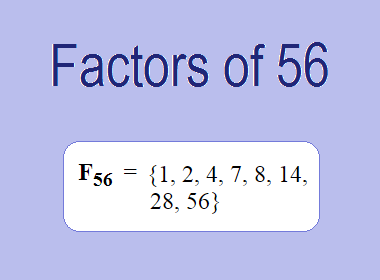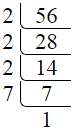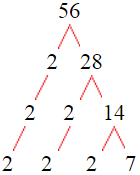# Factors of 56The factors of 56 are 1, 2, 4, 7, 8, 14, 28, and 56 i.e. F56 = {1, 2, 4, 7, 8, 14, 28, 56}. The factors of 56 are all the numbers that can divide 56 without leaving a remainder.

We can check if these numbers are factors of 56 by dividing 56 by each of them. If the result is a whole number, then the number is a factor of 56. Let's do this for each of the numbers listed above:

·        1 is a factor of 56 because 56 divided by 1 is 56.

·        2 is a factor of 56 because 56 divided by 2 is 28.

·        4 is a factor of 56 because 56 divided by 4 is 14.

·        7 is a factor of 56 because 56 divided by 7 is 8.

·        8 is a factor of 56 because 56 divided by 8 is 7.

·        14 is a factor of 56 because 56 divided by 14 is 4.

·        28 is a factor of 56 because 56 divided by 28 is 2.

·        56 is a factor of 56 because 56 divided by 56 is 1.

## How to Find Factors of 56?

1 and the number itself are the factors of every number. So, 1 and 56 are two factors of 56. To find the other factors of 56, we can start by dividing 56 by the numbers between 1 and 56. If we divide 56 by 2, we get a remainder of 0. Therefore, 2 is a factor of 56. If we divide 56 by 3, we get a remainder of 2. Therefore, 3 is not a factor of 56.

Next, we can check if 4 is a factor of 56. If we divide 56 by 4, we get a remainder of 0. Therefore, 4 is a factor of 56. We can continue this process for all the possible factors of 56.

Through this process, we can find that the factors of 56 are 1, 2, 4, 7, 8, 14, 28, and 56. These are the only numbers that can divide 56 without leaving a remainder.

********************

********************

## Properties of the Factors of 56

The factors of 56 have some interesting properties. One of the properties is that the sum of the factors of 56 is equal to 120. We can see this by adding all the factors of 56 together:

1 + 2 + 4 + 7 + 8 + 14 + 28 + 56 = 120

Another property of the factors of 56 is that the prime factors of 56 are 2 and 7 only.

## Applications of the Factors of 56

The factors of 56 have several applications in mathematics. One of the applications is in finding the highest common factor (HCF) of two or more numbers. The HCF is the largest factor that two or more numbers have in common. For example, to find the HCF of 56 and 42, we need to find the factors of both numbers and identify the largest factor they have in common. The factors of 56 are 1, 2, 4, 7, 8, 14, 28, and 56. The factors of 42 are 1, 2, 3, 6, 7, 14, 21, and 42. The largest factor that they have in common is 14. Therefore, the HCF of 56 and 42 is 14.

Another application of the factors of 56 is in prime factorization. Prime factorization is the process of expressing a number as the product of its prime factors. The prime factors of 56 are 2 and 7. We can express 56 as:

56 = 2 × 2 × 2 × 7

We can do prime factorization by division and factor tree method also. Here is the prime factorization of 56 by division method,56 = 2 × 2 × 2 × 7

Here is the prime factorization of 56 by the factor tree method,56 = 2 × 2 × 2 × 7

## Conclusion

The factors of 56 are the numbers that can divide 56 without leaving a remainder. The factors of 56 are 1, 2, 4, 7, 8, 14, 28, and 56. The factors of 56 have some interesting properties, such as having a sum of 120. The factors of 56 have several applications in mathematics, such as finding the highest common factor and prime factorization.